# Adding Average GCSE Scores for KS5 imports in England

The Prior Attainment Article gives further detail of the prior attainment scores we use, and the information required to calculate the scores.

The prior attainment is crucial to your Alps analysis as it provides the baseline score from which we can measure your students’ progress. Without this baseline, we won’t be able to provide value-add analysis.

The following import steps are specifically for KS5 students in England:

If you did not import your prior attainment in the Year Group import or you need to calculate the Prior Attainment, please click on + Add Data to launch the Import Prior Attainment wizard.Select 'Use my own GCSE Scores'.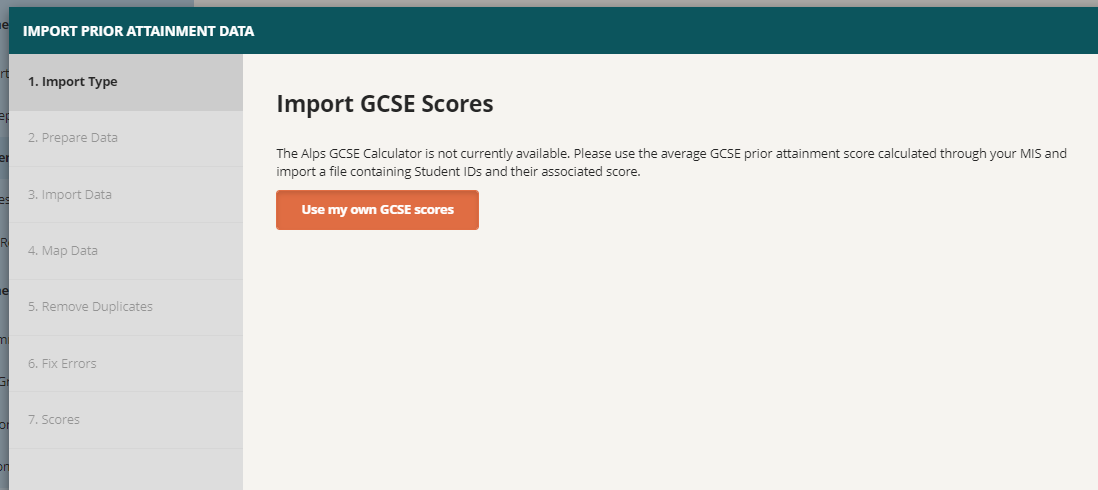Calculating your scores without short courses:

If you do not have any short course GCSEs, please follow these steps when calculating your scores:
1. For English and International schools following the reformed curriculum, the 9-1 grades for the full course GCSEs are scored respectively.
2. For English and International schools using A*-G grades, here are what the grades are worth for the full course GCSEs: A* = 8.5, A = 7, B = 5.5, C = 4, D = 3, E = 2, F = 1.5, G = 1, U = 0.
3. For any A*-G grades that you have in your Excel file, we recommend that you use the Find and Replace (ctrl + F) tool to quickly convert the letter grades to their number equivalents.
4. Please treat the Combined Science grades as two separate grades in your calculation, by splitting them out over two separate columns in Excel.
5. Exclude any BTEC/vocational courses from your calculation.
Please format your file like a Marksheet, with your GCSE Subjects across the top of the file and your Student IDs down the left hand side of the file: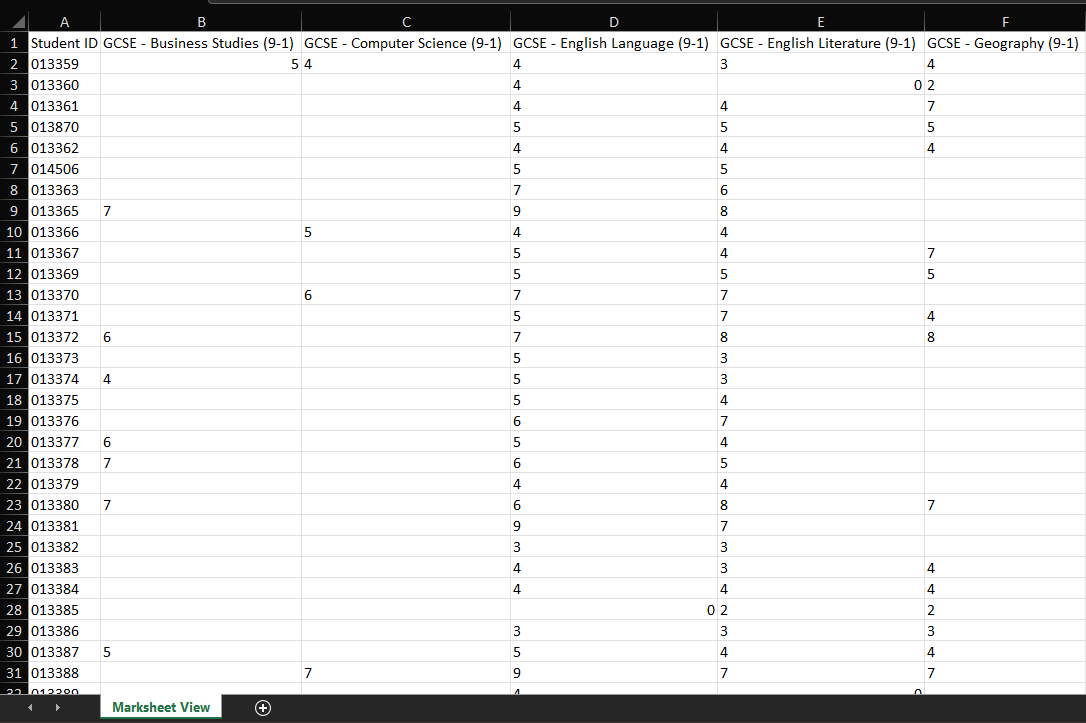Then, simply use an Average Formula in Excel to calculate the scores: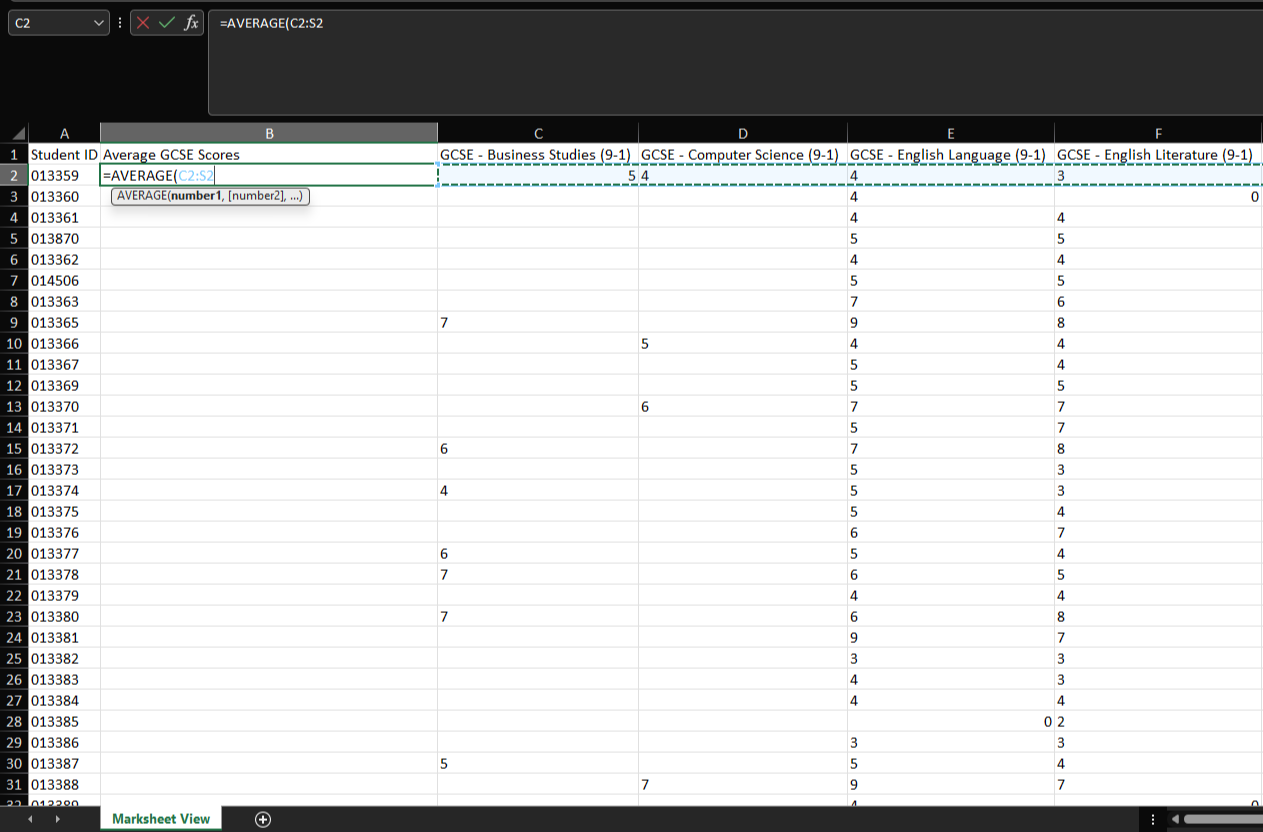Once the scores have been calculated, all you then need to import is the Student ID and the Average GCSE Score: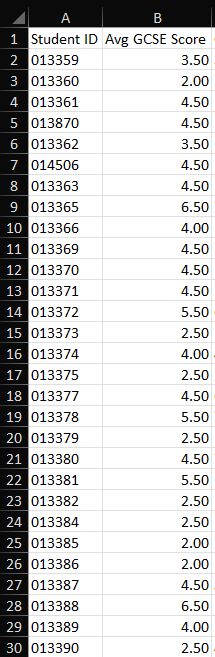Calculating your scores with short courses:

If you have any short course GCSEs, please follow these steps when calculating your scores:
1. For English and International schools following the reformed curriculum, the 9-1 grades for the full course GCSEs are scored respectively. The short courses are worth half. (9 = 4.5, 8 = 4 etc.)
2. For English and International schools using A*-G grades, here are what the grades are worth for the full course GCSEs: A* = 8.5, A = 7, B = 5.5, C = 4, D = 3, E = 2, F = 1.5, G = 1, U = 0. The short courses are worth half (A* = 4.25, A = 3.5 etc.)
3. For any A*-G grades that you have in your Excel file, we recommend that you use the Find and Replace (ctrl + F) tool to quickly convert the letter grades to their number equivalents.
4. Please treat the Combined Science grades as two separate grades in your calculation, by splitting them out over two separate columns in Excel.
5. Exclude any BTEC/vocational courses from your calculation.
When calculating your scores with short courses you will need to create an Equivalence row at the bottom of your Marksheet to count how many GCSEs each subject is worth. A full course is worth 1 and a short course is worth 0.5, as shown in this example: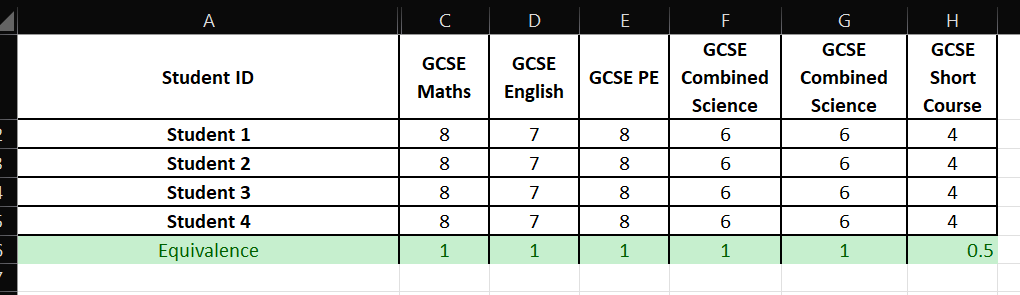The calculation you need to use is as follows:

Total sum of points divided by GCSE equivalence.

In the example below, I have 5 full courses and 1 short course, which is equal to 5.5 GCSEs.

My total points are 39.

So, I need to divide 39 by 5.5, which comes out at 7.09.

See example below: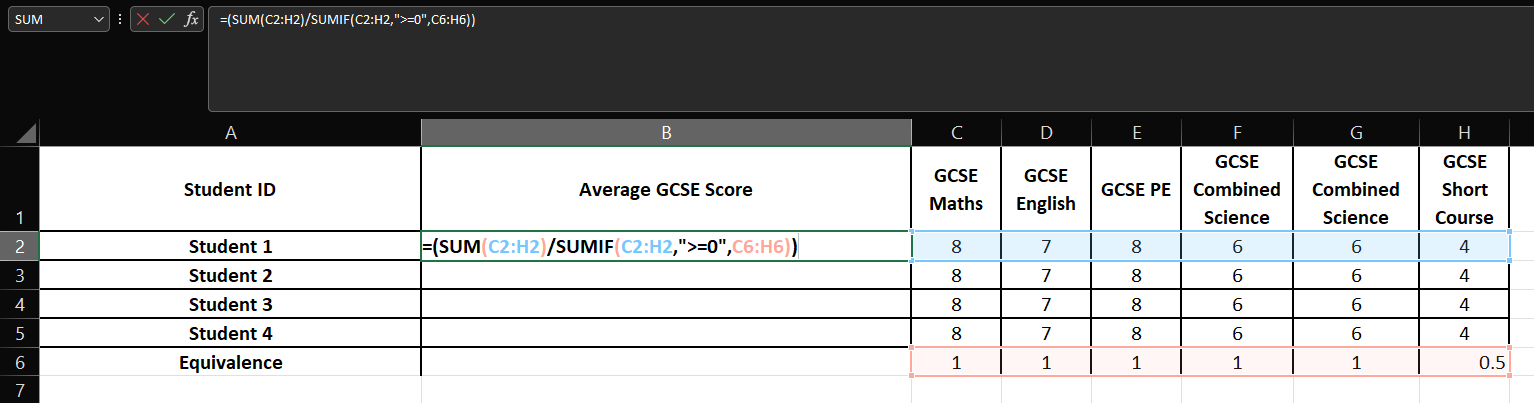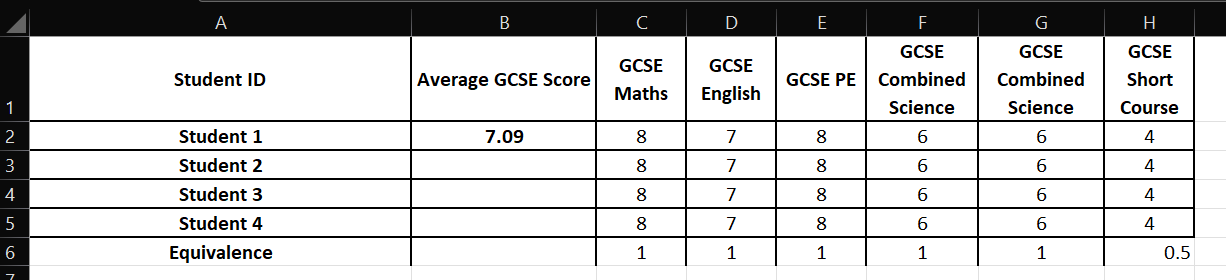If you need to import additional data, including prior attainment, please go to Students, use the drop-down lists to navigate to your Academic Year and Year Group, then click on Import Additional Data. Choose Update and merge, shown below. You can then launch the import wizards from this screen. This applies for all Key Stages in all countries.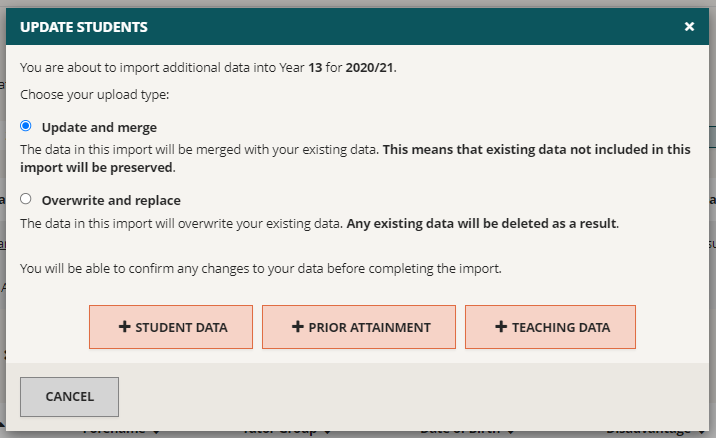Once you have imported your students’ prior attainment, you will be able to import personalised targets. This process is very similar to the other grades imports, and is covered in more detail in the Personalised Targets Article.